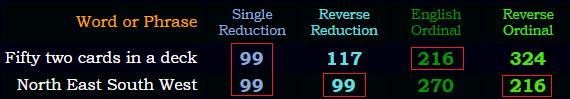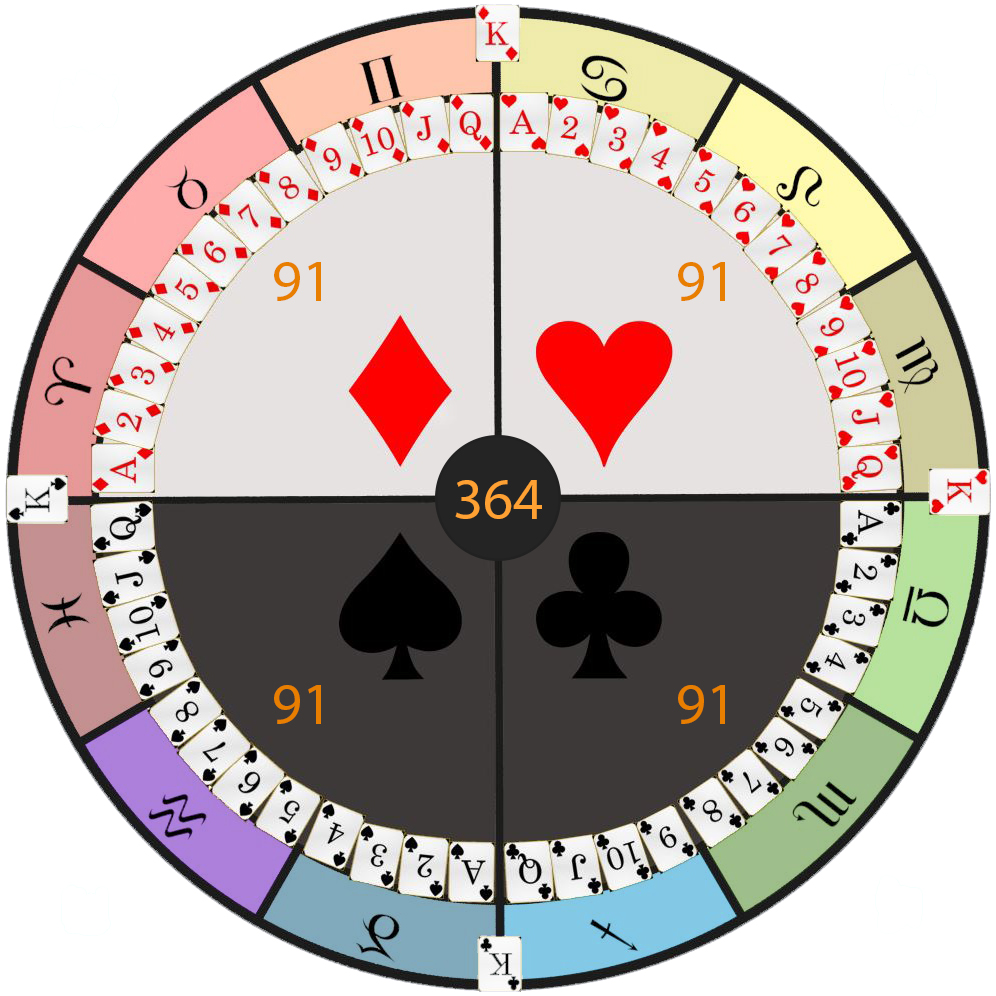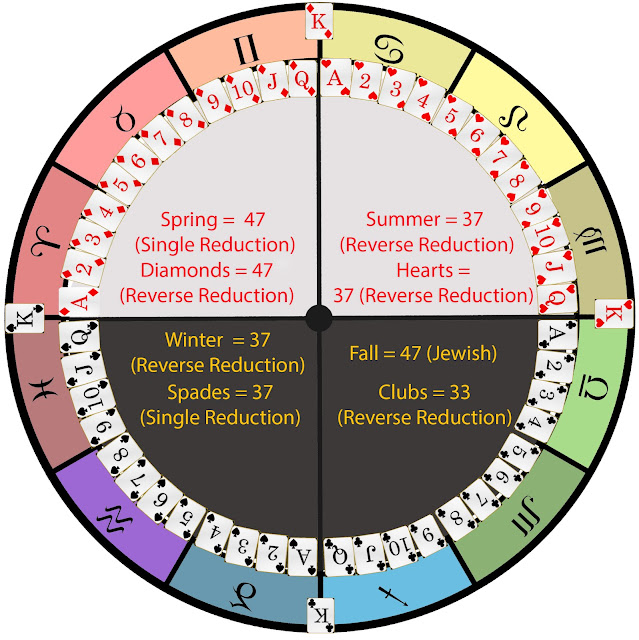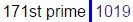## Cipher## May 8, 2017

### Deck of cards (Calendar) and Poker terms

I played Poker for a couple of years and I'm not sure why I didn't think of gematrinating some of this stuff earlier but the game of Poker has some good numbers popping up

Out of 52 playing cards that are used today we can see a clear connection to 4 Suits of Tarot Decks but people can't exactly agree on which symbols correspond to the modern playing cards so I'll skip dealing with the Tarot to make things very simple here.

The first thing you notice when you look at a deck of cards is that you have 2 colors - Red = 9+4+5 = 18 (Reverse Reduction)Black = 7+6+8+6+7 = 34 (Reverse Reduction), so red+black = 18+34 = 52 cards, red cards stand for the hot months of the year and the black ones for cold
There are 52 weeks in a year and both "Earth" and "Card game" have matching gematria
Earth = 5+1+18+20+8 = 52 (English Ordinal) (25 full reduction)
card game = 3+1+18+4 + 7+1+13+5 = 52 (English Ordinal)
Poker = 2+3+7+4+9 = 25 (Reverse Reduction)
bluff = 7+6+6+3+3 = 25 (Reverse Reduction)The 2nd thing we see is 4 suits of 13 cards standing for "Four seasons" = 6+6+3+9 + 1+5+1+1+6+5+1 = 44 (Full Reduction), Deck of Cards = 4+5+3+2 + 6+6 + 3+1+9+4+1 = 44 (Full Reduction)Clock = 3+12+15+3+11 = 44 (English Ordinal) I find interesting that 52 is 44 in base 12 numbering system

In the deck we have 12 Face cards (Jacks, Queens, Kings) connecting to 12 months of the year, if you put each of the 12 face cards on each of the months and add up their values it sums to 144 - (11+12+13) x 4 seasons/suits = 144, Time = 100+9+30+5 = 144 (Jewish), Face cards = 6+1+3+5 + 3+1+80+4+90 = 193 (Jewish) <-- 44th prime number
13 cards in a suit is also connected to the Lunar Calendar

So here is how I laid out the cards over the calendar, it might not be 100% correct with the arrangement of the symbols I chose for the seasons but I was simply going with what made the most sense to meSo we have 4 suits of 13 cards and the 13th triangular number is 91 which is the length of a season in days (4x91 gives us 364 days of the year), Ninety one = 4+9+4+4+7+2 + 3+4+4 = 41 (Reverse Reduction), 13th triangular number written out sums to 41 (the 13th prime)
"Game of Poker" = 7+1+40+5 + 60+6 + 70+60+20+5+90 = 364 (English Extended)

Also it's important to notice that Kings fall on the 4 Cardinal points which is spelled "Kardinal" in many European languages, we also see 4 Kings mentioned in the Bible which encodes astrology all the way through

In the picture below you can see some correspondence between the opposing seasonsAlso Diamonds corresponds to Clubs through 33, Diamonds = 23+18+26+14+12+13+23+8 = 137 (Reverse Ordinal) <- 33rd prime number
Diamond = 4+9+1+4+6+5+4 = 33 (Full Reduction)

Here are some terms that come up when you're dealing with card games and mostly poker
All in = 8+6+6 + 9+4 = 33 (Reverse Reduction)
dealer button = 4+5+1+12+5+18 + 2+21+20+20+15+14 = 137 (English Ordinal)
"2" cards are also known as "Deuces" = 5+4+6+6+4+8 = 33 (Reverse Reduction)
"3" cards are also known as "Treys" = 2+9+5+7+10 = 33 (Single Reduction)
In Poker you can often hear "Two of a kind", "Three of a kind" or "Four of a kind" so if you leave out the number it also sums to 33 - "of a kind" = 6+6 + 1 + 2+9+5+4 = 33 (Full Reduction)
"Face cards" = 6+1+3+5 + 3+1+9+4+1 = 33 (Full Reduction)
"playing cards" = 7+3+1+7+9+5+7 + 3+1+9+4+10 = 66 (Single Reduction)
"Flush" = 6+12+21+19+8 = 66 (English Ordinal) - "Thirty three" = 2+8+9+9+2+7 + 2+8+9+5+5 = 66 (Full Reduction)
The strongest hand in Texas Hold'em is called "Royal flush" = 18+15+25+1+12 + 6+12+21+19+8 = 137 (English Ordinal)

"Poker Game" = 2+3+7+4+9 + 2+8+5+4 = 44 (Reverse Reduction)
"Pair" = 16+1+9+18 = 44 (English Ordinal)
"Call" = 3+1+20+20 = 44 (Jewish)
"Small Blind" = 10+4+1+3+3 + 2+3+9+5+4 = 44 (Single Reduction)

Another name for "Three of a kind" is "Triplets" = 20+18+9+16+12+5+20+19 = 119 (English Ordinal)
"Full house" = 6+21+12+12 + 8+15+21+19+5 = 119 (English Ordinal)
Here's something interesting connecting to WSOPNovember Nine? 11/9? Notice how "World Series of Poker" sums to 116 and you can easily flip that into 911
"World Series of Poker" = 5+6+9+3+4 + 10+5+9+9+5+10 + 6+6 + 7+6+2+5+9 = 116 (Single Reduction)
"Texas Hold'em" = 7+22+3+26+8 + 19+12+15+23 + 22+14 = 171 (Reverse Ordinal)#### 1 comment:

1.This is great! Why did you stop decoding? You have to decode more. Your decodes are outstanding.